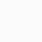# Electric Field Due to a Point Charge

Chapter-1|Electric Charges and Fields | NCERT 12th Physics:

Electric Field Due to a Point Charge:

Let a point charge q is placed at the origin O. We wish to determine it’s electric field at a point P, such that op=r.

Now if we placed a test charge q₀ at point P, then according to coulomb’s law the force on q₀ is

If E is the electric field at a point P due to charge +q at origin, then

From the above formula it is clear that the electric field at any point in space due to a charge depends only on the distance.

## 1 thought on “Electric Field Due to a Point Charge”

1.Harishankar says:

Thanku